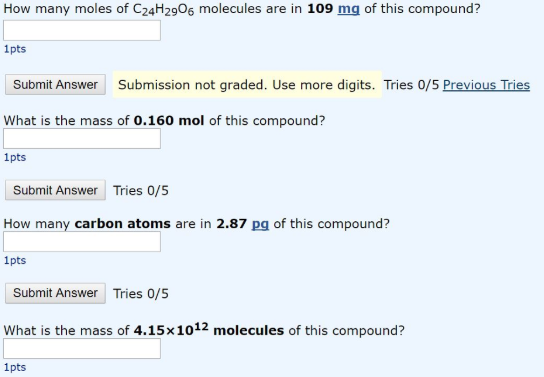# Problem: How many moles of C24H29O6 molecules are in 109 mg of this compound? What is the mass of 0.160 mol of this compound? How many carbon atoms are in 2.87 pg of this compound?What is the mass of 4.15 x 1012 molecules of this compound?

###### FREE Expert Solution
97% (401 ratings)###### Problem Details

How many moles of C24H29O6 molecules are in 109 mg of this compound?

What is the mass of 0.160 mol of this compound?

How many carbon atoms are in 2.87 pg of this compound?

What is the mass of 4.15 x 1012 molecules of this compound?